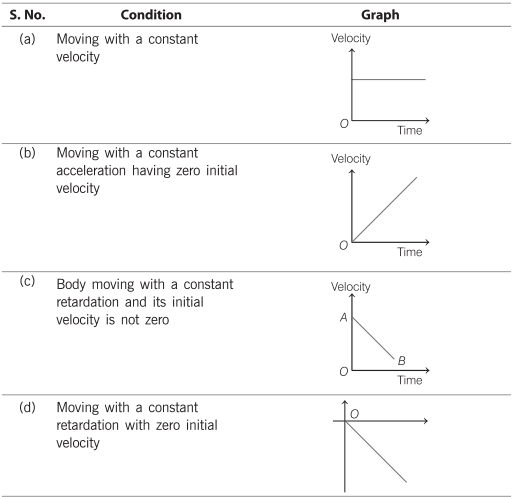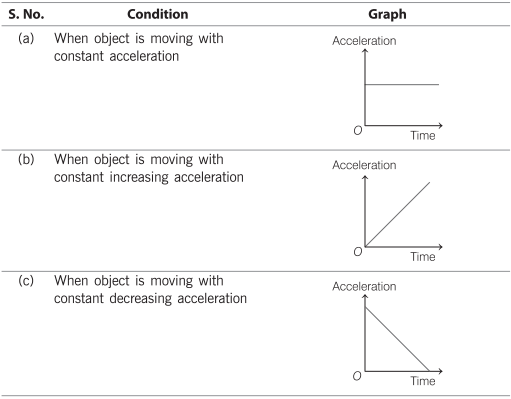# Graphs of Motion in a Straight Line | Displacement, Velocity, Acceleration Time Graphs

## Graphs of Motion in a Straight Line | Displacement, Velocity, Acceleration Time Graphs

We are giving a detailed and clear sheet on all Physics Notes that are very useful to understand the Basic Physics Concepts.

Types of Graphs of Motion Physics:
Displacement Time GraphNote: Slope of displacement-time graph gives average velocity.

Velocity Time GraphNote: Slope of velocity-time graph gives average acceleration.

Acceleration Time GraphMotion in a Straight Line Topics: